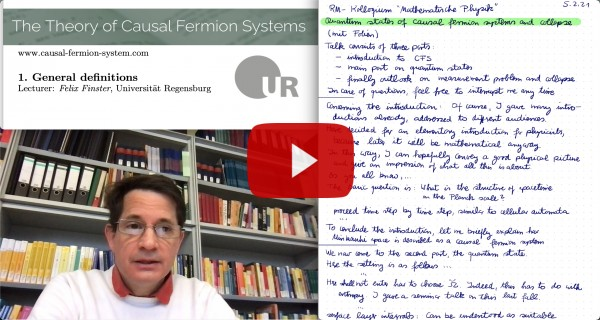# The Theory of Causal Fermion Systems

## Connection to General RelativityIn [cfs16, Chapter 4] it is shown that in the continuum limit, the Euler-Lagrange equations give rise to the Einstein equations, up to possible higher order corrections in curvature (which scale in powers of $(\delta^2\:\text{Riem})$, where $\delta$ is the Planck length and $\text{Riem}$ is the curvature tensor). In this limiting case, spacetime goes over to a Lorentzian manifold. The gravitational coupling constant $G \sim \delta^2$ is determined by the length scale $\delta$ of the microscopic spacetime structure.

The procedure in [cfs16, Chapter 4] is to analyze the continuum limit for linearized gravity. The results are then combined with the general covariance with the approach and the scaling behavior of the light-cone expansion. Here we only mention two specific features:

• As is clear already from dimensional arguments, compared to the matter contribution, the contribution involving the Ricci tensor involves a prefactor of dimension one over length squared. The resulting length scale $\delta$ is related to the regularization of the kernel fermionic projector. More precisely, $\delta$ is the length scale at which the chiral symmetry in the neutrino sector is broken. This becomes clear in the formalism of the continuum limit by the appearance of so-called shear and general surface states.
• When evaluating the Euler-Lagrange equations of the causal action principle in the continuum limit, one gets the Einstein equations only in the form where both tensor indices contracted with a lightlike vector $\xi$, i.e.

$\Big( R_{jk} – \frac{1}{2}\: R\: g_{jk} – \kappa \,T_{jk} \Big) \: \xi^j \xi^k = 0$

(for details see [cfs16, Section 4.9]). Clearly, the cosmological term drops out in this evaluation. It could in principle be determined by analyzing the continuum limit to lower degree on the light cone, but this analysis has not yet been carried out.

This derivation of the Einstein equations  has two disadvantages. First, it is rather technical, because it relies on the detailed form of the regularized light-cone expansion of the kernel of the fermionic projector. Consequently, the derivation does not give a good intuitive understanding of the underlying mechanisms. Second and more importantly, the Einstein equations are recovered only in the continuum limit, but the methods do not give any insight into the geometric meaning of the Euler-Lagrange equations for more general “quantum” spacetimes.

In view of theses disadvantages, it is an important task to study the nature of the gravitational interaction as described by the causal action principle without referring to limiting cases, but instead by analyzing directly the corresponding EL equations. We now outline different approaches in this direction.

## Two-Dimensional Area and Matter Flux

In [CFI19] the notions of two-dimensional area, Killing fields and matter flux are introduced in the setting of causal fermion systems. It is shown that for critical points of the causal action, the area change of two-dimensional surfaces under a Killing flow in null directions is proportional to the matter flux through these surfaces. This relation generalizes an equation in classical general relativity due to Ted Jacobson to the setting of causal fermion systems.

## A Positive Mass Theorem for Static Causal Fermion Systems

In [pmt19] a positive mass theorem is proven for asymptotically flat, static causal fermion systems. The total mass is defined as a limit of surface layer integrals which compare the measures describing the asymptotically flat spacetime and a vacuum spacetime near spatial infinity. More precisely, denoting the measures by $d\tilde{\rho} = dt \,d\tilde{\mu}$ and $d\rho=dt\, d\mu$, respectively, the total mass $\mathfrak{M}$ is defined by

$\displaystyle \mathfrak{M} = \lim_{\Omega_n \nearrow N, \; \tilde{\Omega}_n \nearrow \tilde{N} }$

$\displaystyle \times \bigg( \int_{\tilde{\Omega}_n} \!\!d\tilde{\mu}(x) \int_{N\setminus \Omega_n} \!\!\!\!\!\!\!\!d\mu(y)\: \L_\kappa(x,y) – \int_{\Omega_n} \!\!d\mu(x) \int_{\tilde{N} \setminus \tilde{\Omega}_n} \!\!\!\!\!\!\!\!d\tilde{\mu}(y)\: \L_\kappa(x,y) \bigg)$ ,

where the sets $\Omega_n$ and $\tilde{\Omega}_m$ must have the same volume, i.e. $\mu(\Omega_n)=\tilde{\mu}(\tilde{\Omega}_n)$. It is shown that for Dirac sea vacua defined in the Schwarzschild geometry, this definition reduces to the ADM mass. Moreover, it is shown that, if a local energy condition holds, then the total mass is non-negative.

There is a video of a seminar talk on the positive mass theorem given at Universität Tübingen in July 2020. Moreover, the review article [grossmann21] deals with the connection to gravity.